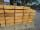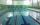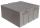# How much

How much money will we pay for 20 planks 4m long, 15cm wide and 26mm thick when 1m³ of wood costs 4500kč?

Result

x =  1404 Kc

#### Solution:Leave us a comment of example and its solution (i.e. if it is still somewhat unclear...):

Showing 0 comments:Be the first to comment!#### To solve this example are needed these knowledge from mathematics:

Do you want to convert length units? Do you know the volume and unit volume, and want to convert volume units?

## Next similar examples:

1. Wood planks1 m3 of wood planks costs 179 EUR. How much will I pay for 11 planks measuring 6 cm, 10 cm and 6 m?
2. AquariumAquarium is cube with edge 45 cm. How much water can fit in there?
3. Pool 3How long will fill pool cuboid shape (8m 6m 1.5m) when flows 15 liters/s?
4. Water tankWater tank shape of cuboid has dimensions of the bottom 7.5 meters and 3 meters. How high will reach the water in the tank will flow 10 liters of water per second and the inflow will be open 5/6 hour? (Calculate to one decimal place and the results give in
5. WaterInto a full cylindrical tank high 3.6 with base radius 2.3 m we insert cuboid with dimensions 0.5 m, 1.9 m, 0.7 m. How many liters of water will come out?
6. Pool in litresPool has a width of 3.5 m length of 6 m and a height 1.60 meters. Calculate pool volume in liters.
7. Fire tankHow deep is the fire tank with the dimensions of the bottom 7m and 12m, when filled with 420 m3 of water?
8. Cube cornersThe wooden cube with edge 64 cm was cut in 3 corners of cube with edge 4 cm. How many cubes of edge 4 cm can be even cut?
9. Cylindrical tank 2If a cylindrical tank with volume is used 12320cm raised to the power of 3 and base 28cm is used to store water. How many liters of water can it hold?
10. HectolitersHow many hectoliters of water fits into cuboid tank with dimensions of a = 3.5 m b = 2.5 m c = 1.4 m?
11. Two cuboidsFind the volume of cuboidal box whose one edge is: a) 1.4m and b) 2.1dm
12. AquariumAquarium is rectangular box with square base containing 76 liters of water. Length of base edge is 42 cm. To what height the water level goes?
13. Customary lengthConvert length 65yd 2 ft to ft
14. The shopThe shop has 3 hectoliters of water. How many liter bottles is it?
15. Water reservoirThe water tank has a cuboid with edges a= 1 m, b=2 m , c = 1 m. Calculate how many centimeters of water level falls, if we fill fifteen 12 liters cans.
16. Volume of cubeSolve the volume of a cube with width 26cm .
17. Concrete tankHow many m3 of concrete is in the tank shape of a cuboid with dimensions of 2000 cm and 5.6 meters and 70 dm, if the concrete takes up 3/7 of the tank?